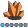qkaiwei
Calcite | Level 5

## Proc optmodel - Dynamic variable and objective function

Hi all:

I define 4 numeric variables: a, b, c, d and want to calculate the minimal value of objective function, and meet a problem.

When interating, the a variable named flag is based on variable a, b : flag=max(a,b), and the flag variable is also involved in the objective function, for example:

min f= X + a*Y * flag - ........;

that means the flag variable value and the objective function will change during each interation. So how to define them in proc optmodel.

Thanks!

1 ACCEPTED SOLUTION

Accepted Solutionsqkaiwei
Calcite | Level 5

## Re: Proc optmodel - Dynamic variable and objective function

Use PROC FCMP to create some functions to solve it, the mechanism of SAS is a little different with that of MATLAB.qkaiwei
Calcite | Level 5